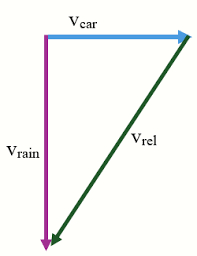Raindrops

The car runs on a horizontal track at a constant speed of 20 m2-1. It is raining. Raindrops fall in a vertical direction at a speed of 6 m/s.
a) How fast is the speed of the drops relative to the car windows?
b) What is the angle of the raindrops on the car window with the vertical direction?

v =  20.8806 m/s
α =  73.3008 °

Step-by-step explanation:Did you find an error or inaccuracy? Feel free to write us. Thank you!Tips to related online calculators
Our vector sum calculator can add two vectors given by their magnitudes and by included angle.
Do you want to convert velocity (speed) units?